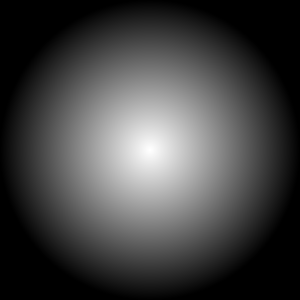# How to draw conic gradient?

Hi !

Does anyone know how to achieve this kind of gradient ? (the gradient part, not the mask part)Thanks !

http://www.juce.com/doc/classGraphics#a57478bc2496ebb84696e5ba64b455965

HTH

Hi Daniel, thanks for helping :)

I dont want a radial gradient,  I want a linear one, but with a circular shape.

I want this:not this:Yes ... seems tricky even in an SVG file :/

It doesn't look like JUCE has anything for Conical Gradients.  You might be able to find something on the Web on how you can make one, or how to make something like a color wheel if that's what you're after.

The only way I can think of doing it in JUCE is by brute force. Convert the X,Y coordinates to radians and in a loop create small gradients and draw the shape piece by piece.

Rail

"Conic Gradient", thats the keyword I was missing, thanks !

I'll try to derive a ColourConicalGradient class from ColourGradient, thanks everyone !

http://www.juce.com/forum/topic/photoshop-layer-effects-juce-controls?page=1

You might be able to use it to implement what you're wanting, if it's still working. Take a look at the "angle gradient" in that post. It looks like a conical gradient to me.

If you look at the GradientOverlay image, it's doing pretty much exactly what you're wanting.

Bumping a very old thread, does anyone have a modern way to do that?

I’ve got some code somewhere that does this.
I’ll try and dig it out tomorrow.

2 Likes

Here you go. It’s not the fastest implementation as it uses an intermediate image and fills every pixel but should work ok:

``````//==============================================================================
The gradient will start at -Pi radians (or 90 degrees to the left) and continue all the way round.
*/
{
Image img (Image::ARGB, bounds.getWidth(), bounds.getHeight(), false);
const Image::BitmapData destData (img, Image::BitmapData::writeOnly);

const float cx = (float) bounds.getCentreX();
const float cy = (float) bounds.getCentreY();

const float scale = (1.0f / (2.0f * MathConstants<float>::pi));

HeapBlock<PixelARGB> lookup;
const int numEntries = cg.createLookupTable (AffineTransform(), lookup);

for (int y = 0; y < bounds.getHeight(); ++y)
{
for (int x = 0; x < bounds.getWidth(); ++x)
{
const float angleRadians = FastMathApproximations::atan2 (bounds.getY() + y - cy, bounds.getX() + x - cx);
const float a = scale * (angleRadians + MathConstants<float>::pi);
const int index = roundToInt (a * (numEntries - 1));
jassert (isPositiveAndNotGreaterThan (a, 1.0f));
jassert (isPositiveAndBelow (index, numEntries));

destData.setPixelColour (x, y, lookup[index]);
}
}

g.drawImageAt (img, bounds.getX(), bounds.getY());
}
``````
9 Likes

I’ve created a pull request to add a conical gradient pattern to the `ColourGradient` class:

Here’s an example……from the following code:

``````void MainComponent::paint (juce::Graphics& g)
{
const auto centre = getLocalBounds().toFloat().getCentre();
const auto pi = juce::MathConstants<float>::pi;

juce::Colours::yellow, centre.withY (centre.y + 100.f),
false, // We don't want a radial pattern
true); // We DO want a conical pattern

juce::Path p;
p.addCentredArc (centre.x, centre.y, 100.f, 100.f, 0.f, -pi * 0.75f, pi * 0.75f, true);
g.strokePath (p, juce::PathStrokeType (50.f));
}
``````
4 Likes

maybe the bool should be an enum to tell which gradient to use (linear, radial, conic) so it would be maybe more future proof (mirror, diamond, …)?